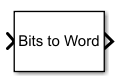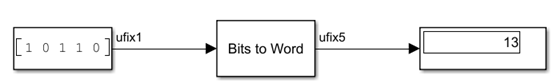# Bits to Word

Convert vector of bits to integer

Since R2023a

•Libraries:
HDL Coder / Logic and Bit Operations

## Description

The Bits to Word block converts a length-N input vector of bits to an N-bit integer. The output of the block is an unsigned integer that has word length N.

The block treats the first element of the input vector as the least significant bit (LSB) of the output and treats subsequent bits as the next significant bits in ascending order. This figure shows the conversion of the bit vector `[1 0 1 1 0]` to the integer value `13`.## Limitations

The block has these limitations:

• The input signal must have the `ufix1` data type.

• Matrix input is not supported.

• Complex input is not supported.

• The input vector length cannot exceed 128.

## Ports

### Input

expand all

Input signal to the block, specified as binary-valued scalar or vector. You can specify an vector containing up to 128 elements.

Example: `[0 1 1 0 0 1 1 1]`

Data Types: `fixdt(0,1,0)`

### Output

expand all

N-bit integer, where N is the size of the input vector, returned as a nonnegative scalar. For example, when you specify a 1-by-8 input signal, then the output signal is an unsigned integer with the `ufix8` data type.

Data Types: `uint8` | `uint16` | `uint32` | `uint64` | `fixed point`

## Version History

Introduced in R2023a